# COUNTIF in Excel - count if not blank, greater than, duplicate or unique

Microsoft Excel provides several functions purposed for counting different kinds of cells, such as blanks or non-blanks, with number, date or text values, containing specific words or character, etc.

In this article, we will focus on the Excel COUNTIF function that is purposed for counting cells with the condition you specify. First, we will briefly cover the syntax and general usage, and then I provide a number of examples and warn about possible quirks when using this function with multiple criteria and specific types of cells.

In essence, COUNTIF formulas are identical in all Excel versions, so you can use the examples from this tutorial in Excel 2016, 2013, 2010 and 2007.

## COUNTIF function in Excel - syntax and usage

Excel COUNTIF function is used for counting cells within a specified range that meet a certain criterion, or condition.

For example, you can write a COUNTIF formula to find out how many cells in your worksheet contain a number greater than or less than the number you specify. Another typical use of COUNTIF in Excel is for counting cells with a specific word or starting with a particular letter(s).

The syntax of the COUNTIF function is very simple:

COUNTIF(range, criteria)

As you see, there are only 2 arguments, both of which are required:

• range - defines one or several cells to count. You put the range in a formula like you usually do in Excel, e.g. A1:A20.
• criteria - defines the condition that tells the function which cells to count. It can be a number, text string, cell reference or expression. For instance, you can use the criteria like these: "10", A2, ">=10", "some text".

And here is the simplest example of Excel COUNTIF function. What you see in the image below is the list of the best tennis players for the last 14 years. The formula `=COUNTIF(C2:C15,"Roger Federer")` counts how many times Roger Federer's name is on the list: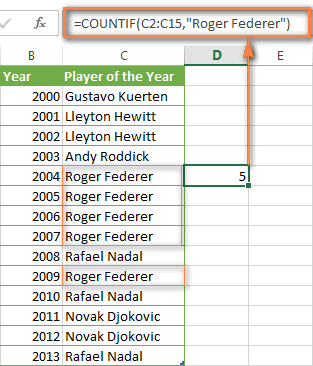Note. A criterion is case insensitive, meaning that if you type "roger federer" as the criteria in the above formula, this will produce the same result.

## Excel COUNTIF function examples

As you have just seen, the syntax of the COUNTIF function is very simple. However, it allows for many possible variations of the criteria, including wildcard characters, the values of other cells, and even other Excel functions. This diversity makes the COUNTIF function really powerful and fit for many tasks, as you will see in the examples that follow.

### COUNTIF formula for text and numbers (exact match)

In fact, we discussed the COUNTIF function that counts text values matching a specified criterion exactly a moment ago. Let me remind you that formula for cells containing an exact string of text: `=COUNTIF(C2:C15,"Roger Federer")`. So, you enter:

• A range as the first parameter;
• A comma as the delimiter;
• A word or several words enclosed in quotes as the criteria.

Instead of typing text, you can use a reference to any cell containing that word or words and get absolutely the same results, e.g. `=COUNTIF(C1:C9,C7)`.

Similarly, COUNTIF formulas work for numbers. As shown in the screenshot below, the formula `=COUNTIF(D2:D9,5)` perfectly counts cells with quantity 5 in Column D.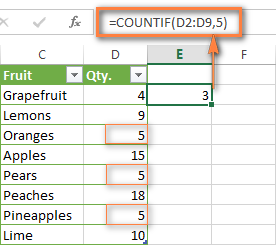### COUNTIF formulas with wildcard characters (partial match)

In case your Excel data include several variations of the keyword(s) you want to count, then you can use a wildcard character to count all the cells containing a certain word, phrase or letters as part of the cell's contents.

Suppose, you have a list of tasks assigned to different persons, and you want to know the number of tasks assigned to Danny Brown. Because Danny's name is written in several different ways, we enter "*Brown*" as the search criteria `=COUNTIF(D2:D10, "*Brown*")`.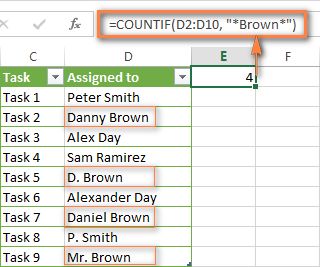An asterisk (*) is used to find cells with any sequence of leading and trailing characters, as illustrated in the above example. If you need to match any single character, enter a question mark (?) instead, as demonstrated below.

Tip. It is also possible to use wildcards with cell references with the help of the concatenation operator (&). For example, instead of supplying "*Brown*" directly in the formula, you can type it in some cell, say F1, and use the following formula to count cells containing "Brown": =COUNTIF(D2:D10, "*"&F1&"*")

#### Count cells beginning or ending with certain characters

You can use either wildcard character, asterisk (*) or question mark (?), with the criterion depending on which exactly result you want to achieve.

If you want to know the number of cells that start or end with certain text no matter how many other characters a cell contains, use these formulas:

`=COUNTIF(C2:C10,"Mr*")` - count cells that begin with "Mr".

`=COUNTIF(C2:C10,"*ed")` - count cells that end with the letters "ed".

The image below demonstrates the second formula in action: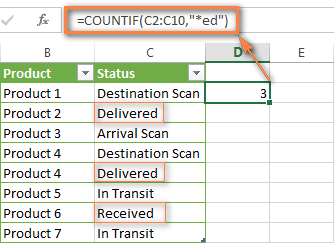If you are looking for a count of cells that start or end with certain letters and contain the exact number of characters, you use the Excel COUNTIF function with the question mark character (?) in the criteria:

`=COUNTIF(D2:D9,"??own")` - counts the number of cells ending with the letters "own" and having exactly 5 characters in cells D2 through D9, including spaces.

`=COUNTIF(D2:D9,"Mr??????")` - counts the number of cells starting with the letters "Mr" and having exactly 8 characters in cells D2 through D9, including spaces.

Tip. To find the number of cells containing an actual question mark or asterisk, type a tilde (~) before the ? or * character in the formula. For example, `=COUNTIF(D2:D9,"*~?*")` will count all cells containing the question mark in the range D2:D9.

### Excel COUNTIF for blank and non-blank cells

These formula examples demonstrate how you can use the COUNTIF function in Excel to count the number of empty or non-empty cells in a specified range.

#### COUNTIF not blank

In some of other Excel COUNTIF tutorials, you may come across formulas for counting non-blank cells in Excel similar to this one:

COUNTIF(range,"*")

But the fact is, the above formula counts only cells containing any text values, meaning that cells with dates and numbers will be treated as blank cells and not included in the count!

If you need a universal COUNTIF formula for counting all non-blank cells in a specified range, here you go:

COUNTIF(range,"<>")

Or

COUNTIF(range,"<>"&"")

This formula works correctly with all value types - text, dates and numbers - as you can see in the screenshot below.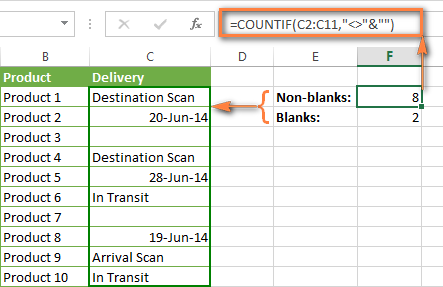#### COUNTIF blank

If you want the opposite, i.e. count blank cells in a certain range, you should adhere to the same approach - use a formula with a wildcard character for text values and with the "" criteria to count all empty cells.

Formula to count cells not containing any text:

COUNTIF(range,"<>"&"*")

Since an asterisk (*) matches any sequence of text characters, the formula counts cells not equal to *, i.e. not containing any text in the specified range.

Universal COUNTIF formula for blanks (all value types):

COUNTIF(range,"")

The above formula correctly handles numbers, dates and text values. For example, here's how you can get the number of empty cells in the range C2:C11:

`=COUNTIF(C2:C11,"")`

Please be aware that Microsoft Excel has another function for counting blank cells, COUNTBLANK. For instance, the following formulas will produce exactly the same results as the COUNTIF formulas you see in the screenshot above:

Count blanks: `=COUNTBLANK(C2:C11)`

Count non-blanks: `=ROWS(C2:C11)*COLUMNS(C2:C11)-COUNTBLANK(C2:C11)`

Also, please keep in mind that both COUNTIF and COUNTBLANK count cells with empty strings that only look blank. If you do not want to treat such cells as blanks, use this formula instead:

`=ROWS(C2:С11)*COLUMNS(C2:С11)-COUNTIF(C2:С11,"<>"&"")`

### COUNTIF greater than, less than or equal to

To count cells with values greater than, less than or equal to the number you specify, you simply add a corresponding operator to the criteria, as shown in the table below.

Please pay attention that in COUNTIF formulas, an operator with a number are always enclosed in quotes.

 Criteria Formula Example Description Count if greater than =COUNTIF(A2:A10,">5") Count cells where value is greater than 5. Count if less than =COUNTIF(A2:A10,"<5") Count cells with values less than 5. Count if equal to =COUNTIF(A2:A10,"=5") Count cells where value is equal to 5. Count if not equal to =COUNTIF(A2:A10,"<>5") Count cells where value is not equal to 5. Count if greater than or equal to =COUNTIF(C2:C8,">=5") Count cells where value is greater than or equal to 5. Count if less than or equal to =COUNTIF(C2:C8,"<=5") Count cells where value is less than or equal to 5.

You can also use all of the above formulas to count cells based on another cell value, you will just need to replace the number in the criteria with a cell reference.

Note. In case of a cell reference, you have to enclose the operator in quotes and add an ampersand (&) before the cell reference. For example, to count cells in the range D2:D9 with values greater than a value in cell D3, you use this formula `=COUNTIF(D2:D9,">"&D3)`: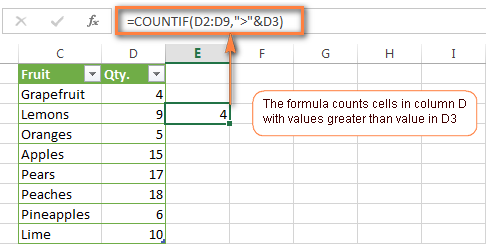If you want to count cells that contain an actual operator as part of the cell's contents, i.e. the characters ">", "<" or "=", then use a wildcard character with the operator in the criteria. Such criteria will be treated as a text string rather than a numeric expression. For example, the formula `=COUNTIF(D2:D9,"*>5*")` will count all cells in the range D2:D9 with contents like this "Delivery >5 days" or ">5 available".

### Using Excel COUNTIF function with dates

If you want to count cells with dates that are greater than, less than or equal to the date you specify or date in another cell, you proceed in the already familiar way using formulas similar to the ones we discussed a moment ago. All of the above formulas work for dates as well as for numbers. Let me give you just a few examples:

 Criteria Formula Example Description Count dates equal to the specified date. =COUNTIF(B2:B10,"6/1/2014") Counts the number of cells in the range B2:B10 with the date 1-Jun-2014. Count dates greater than or equal to another date. =COUNTIF(B2:B10,">=6/1/2014") Count the number of cells in the range B2:B10 with a date greater than or equal to 6/1/2014. Count dates greater than or equal to a date in another cell, minus x days. =COUNTIF(B2:B10,">="&B2-"7") Count the number of cells in the range B2:B10 with a date greater than or equal to the date in B2 minus 7 days.

Apart from these common usages, you can utilize the COUNTIF function in conjunction with specific Excel Date and Time functions such as TODAY() to count cells based on the current date.

 Criteria Formula Example Count dates equal to the current date. =COUNTIF(A2:A10,TODAY()) Count dates prior to the current date, i.e. less than today. =COUNTIF(A2:A10,"<"&TODAY()) Count dates after the current date, i.e. greater than today. =COUNTIF(A2:A10,">"&TODAY()) Count dates that are due in a week. =COUNTIF(A2:A10,"="&TODAY()+7) Count dates in a specific date range. =COUNTIF(B2:B10, ">=6/1/2014")-COUNTIF(B2:B10, ">6/7/2014")

Here is an example of using such formulas on real data (at the moment of writing today was 25-Jun-2014):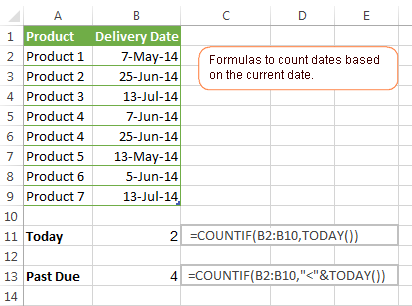### Excel COUNTIF with multiple criteria

In fact, Excel COUNTIF function is not exactly designed to count cells with multiple criteria. In most cases, you'd use its plural counterpart, the COUNTIFS function to count cells that match two or more criteria (AND logic). However, some tasks can be solved by combining two or more COUNTIF functions in one formula.

#### COUNTIF - COUNTIF to count numbers within a range

One of the most common applications of Excel COUNTIF function with 2 criteria is counting numbers within a specific range, i.e. less than X but greater than Y. For example, you can use the following formula to count cells in the range B2:B9 where a value is greater than 5 and less than 15.

`=COUNTIF(B2:B9,">5")-COUNTIF(B2:B9,">=15")`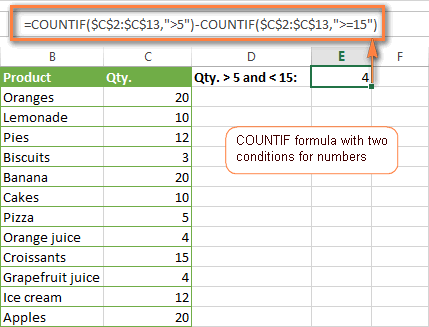#### COUNTIF + COUNTIF to count cells with multiple OR criteria

In situations when you want to get several different items in a range, add 2 or more COUNTIF functions together. Supposing, you have a shopping list and you want to find out how many soft drinks are included. To have it done, use a formula similar to this:

`=COUNTIF(B2:B13,"Lemonade")+COUNTIF(B2:B13,"*juice")`

Please pay attention that we've included the wildcard character (*) in the second criterion, it is used to count all kinds of juice on the list.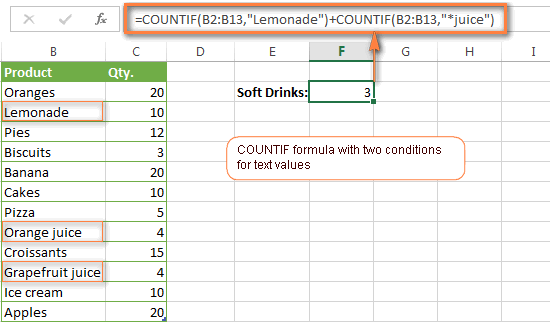In the same manner, you can write a COUNTIF formula with several conditions. Here is an example of the COUNTIF formula with multiple OR conditions that counts lemonade, juice and ice cream:

`=COUNTIF(B2:B13,"Lemonade") + COUNTIF(B2:B13,"*juice") + COUNTIF(B2:B13,"Ice cream")`

For other ways to count cells with OR logic, please see this tutorial: Excel COUNTIF and COUNTIFS with OR conditions.

### Using COUNTIF function to find duplicates and unique values

Another possible usage of the COUNTIF function in Excel is for finding duplicates in one column, between two columns, or in a row.

#### Example 1. Find and count duplicates in 1 column

For example, this simple formula =COUNTIF(B2:B10,B2)>1 will spot all duplicate entries in the range B2:B10 while another function =COUNTIF(B2:B10,TRUE) will tell you how many dupes are there: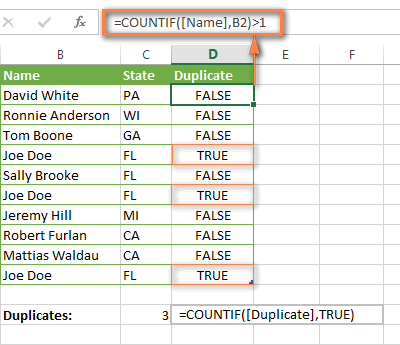#### Example 2. Count duplicates between two columns

If you have two separate lists, say lists of names in columns B and C, and you want to know how many names appear in both columns, you can use Excel COUNTIF in combination with the SUMPRODUCT function to count duplicates:

`=SUMPRODUCT((COUNTIF(B2:B1000,C2:C1000)>0)*(C2:C1000<>""))`

We can even take a step further and count how many unique names there are in Column C, i.e. names that do NOT appear in Column B:

`=SUMPRODUCT((COUNTIF(B2:B1000,C2:C1000)=0)*(C2:C1000<>""))`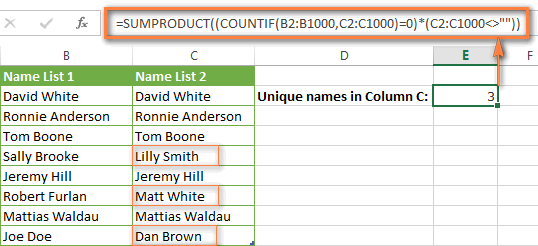Tip. If you want to highlight duplicate cells or entire rows containing duplicate entries, you can create conditional formatting rules based on the COUNTIF formulas, as demonstrated in this tutorial - Excel conditional formatting formulas to highlight duplicates.

#### Example 3. Count duplicates and unique values in a row

If you want to count duplicates or unique values in a certain row rather than a column, use one of the below formulas. These formulas might be helpful, say, to analyze the lottery draw history.

Count duplicates in a row:

`=SUMPRODUCT((COUNTIF(A2:I2,A2:I2)>1)*(A2:I2<>""))`

Count unique values in a row:

`=SUMPRODUCT((COUNTIF(A2:I2,A2:I2)=1)*(A2:I2<>""))`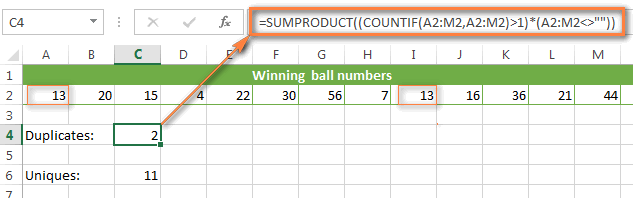## Excel COUNTIF - frequently asked questions and issues

I hope these examples have helped you to get a feel for the Excel COUNTIF function. If you've tried any of the above formulas on your data and were not able to get them to work or are having a problem with the formula you created, please look through the following 5 most common issues. There is a good chance that you will find the answer or a helpful tip there.

#### 1. COUNTIF on a non-contiguous range of cells

Question: How can I use COUNTIF in Excel on a non-contiguous range or a selection of cells?

Answer: Excel COUNTIF does not work on non-adjacent ranges, nor does its syntax allow specifying several individual cells as the first parameter. Instead, you can use a combination of several COUNTIF functions:

Wrong: `=COUNTIF(A2,B3,C4,">0")`

Right: `=COUNTIF(A2,">0") + COUNTIF(B3,">0") + COUNTIF(C4,">0")`

An alternative way is using the INDIRECT function to create an array of ranges. For example, both of the below formulas produce the same result you see in the screenshot:

`=SUM(COUNTIF(INDIRECT({"B2:B8","D2:C8"}),"=0"))`

`=COUNTIF(\$B2:\$B8,0) + COUNTIF(\$C2:\$C8,0)`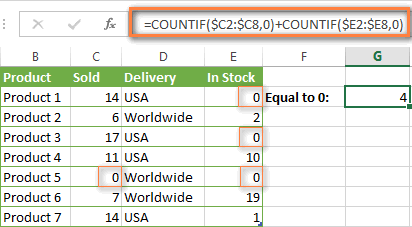#### 2. Ampersand and quotes in COUNTIF formulas

Question: When do I need to use an ampersand in a COUNTIF formula?

Answer: It is probably the trickiest part of the COUNTIF function, which I personally find very confusing. Though if you give it some thought, you'll see the reasoning behind it - an ampersand and quotes are needed to construct a text string for the argument. So, you can adhere to these rules:

If you use a number or a cell reference in the exact match criteria, you need neither ampersand nor quotes. For example:

`=COUNTIF(A1:A10,10)`
or
`=COUNTIF(A1:A10,C1)`

If your criteria includes text, wildcard character or logical operator with a number, enclose it in quotes. For example:

`=COUNTIF(A2:A10,"lemons")`
or
`=COUNTIF(A2:A10,"*")` or `=COUNTIF(A2:A10,">5")`

In case your criteria is an expression with a cell reference or another Excel function, you have to use the quotes ("") to start a text string and ampersand (&) to concatenate and finish the string off. For example:

`=COUNTIF(A2:A10,">"&D2)`
or
`=COUNTIF(A2:A10,"<="&TODAY())`

If you are in doubt whether an ampersand is needed or not, try out both ways. In most cases an ampersand works just fine, e.g. both of the below formulas work equally well.

=COUNTIF(C2:C8,"<=5")
and
=COUNTIF(C2:C8,"<="&5)

#### 3. COUNTIF for formatted (color coded) cells

Question: How do I count cells by fill or font color rather than by values?

Answer: Regrettably, the syntax of the Excel COUNTIF function does not allow using formats as the condition. The only possible way to count or sum cells based on their color is using a macro, or more precisely an Excel User-Defined function. You can find the code working for cells colored manually as well as for conditionally formatted cells in this article - How to count, sum and filter cells by color in Excel.

#### 4. #NAME? error in the COUNTIF formula

Issue: My COUNTIF formula throws a #NAME? error. How can I get it fixed?

Answer: Most likely, you have supplied an incorrect range to the formula. Please check out point 1 above.

#### 5. Excel COUNTIF formula not working

Issue: My COUNTIF formula is not working! What have I done wrong?

Answer: If you have written a formula which is seemingly correct but it does not work or produces a wrong result, start by checking the most obvious things such as a range, conditions, cell references, use of ampersand and quotes.

Be very careful with using spaces in a COUNTIF formula. When creating one of the formulas for this article I was on the verge of pulling my hair out because the correct formula (I knew with certainty it was right!) wouldn't work. As it turned out, the problem was in a measly space somewhere in between, argh... For instance, look at this formula:

`=COUNTIF(B2:B13," Lemonade")`.

At first sight, there is nothing wrong about it, except for an extra space after the opening quotation mark. Microsoft Excel will swallow the formula just fine without an error message, warning or any other indication, assuming you really want to count cells containing the word 'Lemonade' and a leading space.

If you use the COUNTIF function with multiple criteria, split the formula into several pieces and verify each function individually.

And this is all for today. In the next article, we will explore several ways to count cells in Excel with multiple conditions. Hope to see you next week and thanks for reading!

## You may also be interested in

### 736 responses to "COUNTIF in Excel - count if not blank, greater than, duplicate or unique"

1.McKenna says:

Hi there,

For my homework assignment I'm supposed to be using countif for data on earnings for various companies. In one column she wants me to be able to have each value say if it's over \$1billion or below, and if it's below, by how much. I am confused on how to do this in one column. She also wants it to display text of my choosing, which I would say "Above" or "below"

2.Kaushik Sasmal says:

Count in a single formula total A or B Or C
where =COUNTIF(C1,"A") it can count only A

3.Charles says:

The "COUNTIF - COUNTIF to count numbers within a range" section is clearly wrong. If you just manually count the matches on the list, there are a total of 5.

Not sure if your understanding of the function is wrong or just your visual aid, but since I can't get this to work based on your instruction I suspect it is the former.

•Alexander Trifuntov (Ablebits.com Team) says:

Hello Charles!
You're right - the formula does not take into account the number 15. Thank you for your attention. I fixed everything

4.Aamir says:

0100000037 288.00 288.00 PCS TRUE
0100000037 234.00 234.00 PCS TRUE
0100000037 384.00 384.00 PCS TRUE
0100000038 36.00 36.00 PCS TRUE
0100000038 24.00 24.00 PCS TRUE
0100000041 120.00 120.00 PCS TRUE
0100000041 120.00 120.00 PCS TRUE
0100000044 168.00 168.00 PCS TRUE
0100000044 108.00 108.00 PCS TRUE
0100000045 144.00 144.00 PCS TRUE
0100000047 240.00 240.00 PCS TRUE
What formula should i use to count every cell ?
for example 0100000037 this Code is showing in 3 cells so what i ll do to calculate in cell a1=1 a2=2 a3=3 and so and so ?

5.Ravi Sagar says:

I want to count the no of cell with a particular value in continuation and break the counting is that value changes in a cell. which formula I have to use ?
SEGMENT NAME 20Jul 21Jul 22Jul 23Jul 24Jul 25Jul 26Jul 27Jul 28Jul 29Jul Desired count reslut (Count <1)
GOMADBAMANN3 100.00 96.61 76.37 19.06 9.25 0.00 90.24 0.00 0.72 0.00 2
GOMADBAMANN1 100.00 96.07 76.37 20.99 39.14 86.97 99.94 0.00 76.60 0.00 1
GOMADBAMANN2 100.00 96.76 76.37 15.67 0.04 52.55 0.00 82.61 78.17 0.00 1
GOKILKILLIS3 100.00 99.97 100.00 100.00 100.00 97.25 0.00 73.75 33.04 0.00 1
GOKILKILLIS4 100.00 99.97 100.00 100.00 100.00 97.22 0.00 75.06 33.03 0.00 1
GOPARMADHAI1 0.00 36.65 19.06 0.00 0.00 0.00 0.00 0.00 0.00 0.00 7
GOPARMADHAI2 0.00 36.65 19.06 0.00 0.00 0.00 0.00 0.00 0.00 0.00 7
GOPARMADHAI3 0.00 36.65 19.06 0.00 0.00 0.00 0.00 0.00 0.00 0.00 7
GOAMRAMRMAS3 100.00 100.00 100.00 100.00 100.00 0.00 0.00 100.00 34.87 0.00 1
GOJADJADAUD1 0.00 0.00 0.00 0.00 0.00 0.00 0.00 0.00 0.00 0.00 10
GOJADJADAUD2 0.00 0.00 0.00 0.00 0.00 0.00 0.00 0.00 0.00 0.00 10
GOJADJADAUD3 0.00 0.00 0.00 0.00 0.00 0.00 0.00 0.00 0.00 0.00 10
GOHINHINDXX2 99.65 99.24 99.18 99.53 5.48 56.80 99.02 98.91 41.28 0.00 1
GOBELBELA1X1 0.31 0.00 0.00 0.91 0.58 0.11 0.80 2.67 0.00 0.00 2
GOFIRSADABA1 99.74 99.66 99.58 99.85 99.74 0.00 0.00 100.00 100.00 0.00 1
GOFIRSADABA2 99.74 99.65 99.58 99.92 99.74 0.00 0.00 100.00 100.00 0.00 1

6.vijaya says:

Help me, how to calculate duplicate value for below range? i notice when i use this coding, repeated values such as 93 twice, not counted.
=COUNTIFS(Z5:Z14,">=90",Z5:Z14,"<=94")

7.Rizwan says:

I want to count alternate column value. for example i have column A-B-C-D but i need only to count column A & C Value.Please help me to know how i can do by formula.

•Alexander Trifuntov (Ablebits.com Team) says:

Hello!
You did not say by what criteria you will count. Therefore, I cannot give an exact recommendation. However, you can add the two COUNTIF functions. In the first you count by column A, in the second you count by column C. Or you can use the COUNTIFS function. Read more here.

8.Phil Wong says:

How do you use countif when the cells you want to count have a return value in the Dated format example below.
4 Yrs, 11 Mths & 8 Days
The above shows length of service the formula used is:
=IFERROR(IF(E6="","",DATEDIF(E6,\$C\$2,"y") & " Yrs, " & DATEDIF(E6,\$C\$2,"ym") & " Mths & " & DATEDIF(E6,\$C\$2,"md") & " Days "),"")

Now I want to count how many colleagues have a length of service of more that 3 yrs. Is that possible?

Thanks

Phil

•Alexander Trifuntov (Ablebits.com Team) says:

Hello!
You can count the number of dates for which the difference is more than 36 months:

=SUM(--(DATEDIF(\$E\$1:\$E\$10,\$C\$2,"m")>36))

I hope this will help, otherwise please do not hesitate to contact me anytime.

9.Victoria B says:

I have a lengthy list of names with dollars tied to purchases to each of those names. I am attempting to add up the totals for each of the individuals. Some have 3 lines, some have 1 and others have 5. How would I go about getting this to work?

•Alexander Trifuntov (Ablebits.com Team) says:

Hello!
Unfortunately, without seeing your data it hard to give you advice. For me to be able to help you better, please describe your task in more detail. Please specify what you were trying to find, what formula you used and what problem or error occurred. Give an example of the source data and the expected result.
It’ll help me understand it better and find a solution for you.

10.cHARLOTTE says:

what a formula be to count a range of blank cells if filtered.

Ex. have a list, only want to count blank cells. If i only want the blank cells when i filter NY, how can i get it to only count the blank cells when i filter